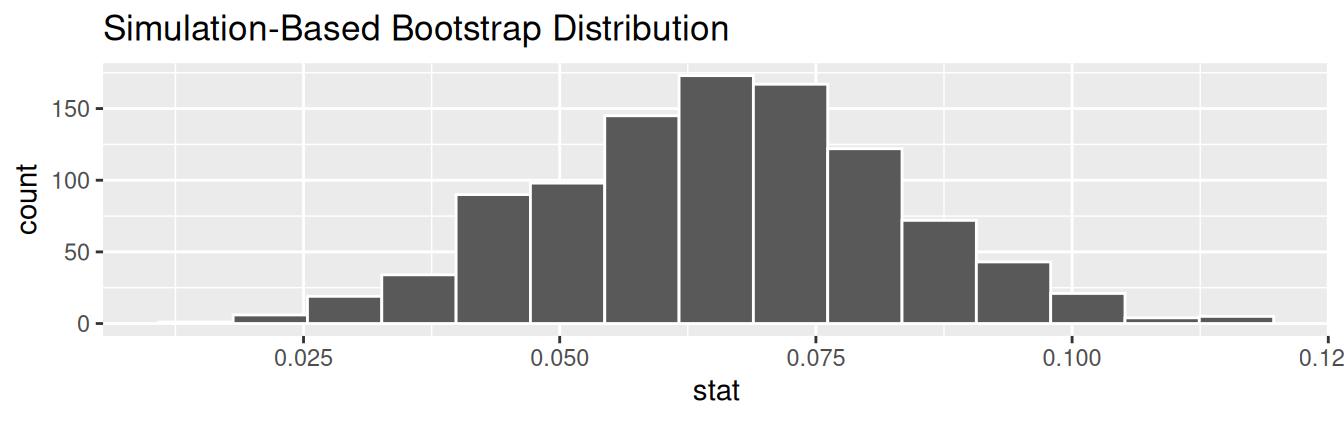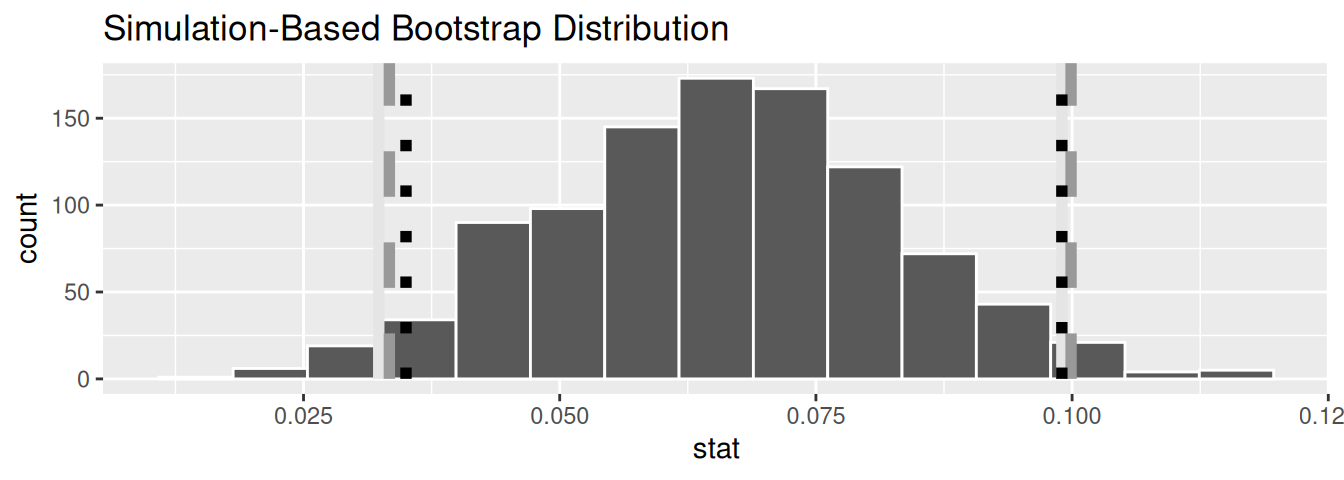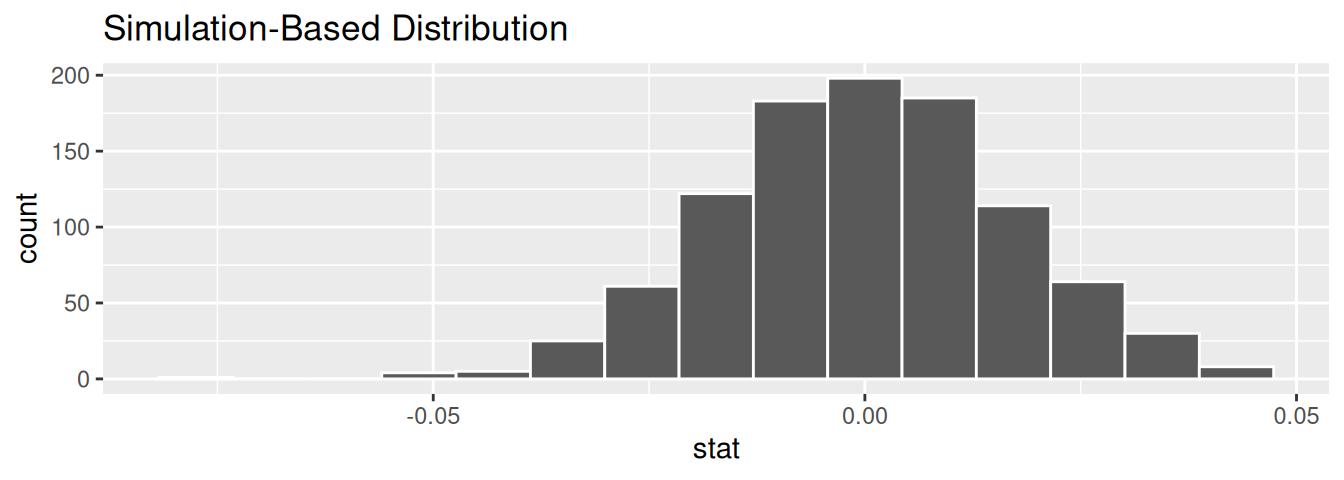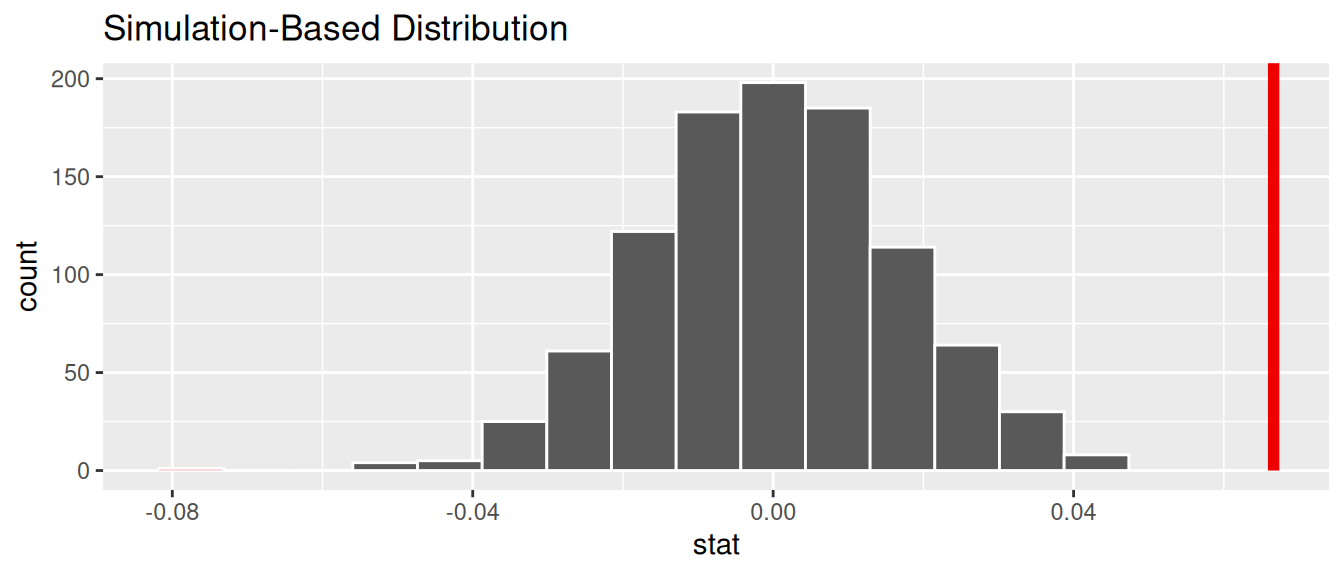## 10.4 Simulation-based inference for regression

Recall in Subsection 10.2.5 when we interpreted the third through seventh columns of a regression table, we stated that R doesn’t do simulations to compute these values. Rather R uses theory-based methods that involve mathematical formulas.

In this section, we’ll use the simulation-based methods you previously learned in Chapters 8 and 9 to recreate the values in the regression table in Table 10.1. In particular, we’ll use the infer package workflow to

• Construct a 95% confidence interval for the population slope $$\beta_1$$ using bootstrap resampling with replacement. We did this previously in Sections 8.4 with the pennies data and 8.6 with the mythbusters_yawn data.
• Conduct a hypothesis test of $$H_0: \beta_1 = 0$$ versus $$H_A: \beta_1 \neq 0$$ using a permutation test. We did this previously in Sections 9.3 with the promotions data and 9.5 with the movies_sample IMDb data.

### 10.4.1 Confidence interval for slope

We’ll construct a 95% confidence interval for $$\beta_1$$ using the infer workflow outlined in Subsection 8.4.2. Specifically, we’ll first construct the bootstrap distribution for the fitted slope $$b_1$$ using our single sample of 463 courses:

1. specify() the variables of interest in evals_ch5 with the formula: score ~ bty_avg.
2. generate() replicates by using bootstrap resampling with replacement from the original sample of 463 courses. We generate reps = 1000 replicates using type = "bootstrap".
3. calculate() the summary statistic of interest: the fitted slope $$b_1$$.

Using this bootstrap distribution, we’ll construct the 95% confidence interval using the percentile method and (if appropriate) the standard error method as well. It is important to note in this case that the bootstrapping with replacement is done row-by-row. Thus, the original pairs of score and bty_avg values are always kept together, but different pairs of score and bty_avg values may be resampled multiple times. The resulting confidence interval will denote a range of plausible values for the unknown population slope $$\beta_1$$ quantifying the relationship between teaching and “beauty” scores for all professors at UT Austin.

Let’s first construct the bootstrap distribution for the fitted slope $$b_1$$:

bootstrap_distn_slope <- evals_ch5 %>%
specify(formula = score ~ bty_avg) %>%
generate(reps = 1000, type = "bootstrap") %>%
calculate(stat = "slope")
bootstrap_distn_slope
# A tibble: 1,000 x 2
replicate      stat
<int>     <dbl>
1         1 0.0651055
2         2 0.0382313
3         3 0.108056
4         4 0.0666601
5         5 0.0715932
6         6 0.0854565
7         7 0.0624868
8         8 0.0412859
9         9 0.0796269
10        10 0.0761299
# … with 990 more rows

Observe how we have 1000 values of the bootstrapped slope $$b_1$$ in the stat column. Let’s visualize the 1000 bootstrapped values in Figure 10.8.

visualize(bootstrap_distn_slope)FIGURE 10.8: Bootstrap distribution of slope.

Observe how the bootstrap distribution is roughly bell-shaped. Recall from Subsection 8.7.1 that the shape of the bootstrap distribution of $$b_1$$ closely approximates the shape of the sampling distribution of $$b_1$$.

#### Percentile-method

First, let’s compute the 95% confidence interval for $$\beta_1$$ using the percentile method. We’ll do so by identifying the 2.5th and 97.5th percentiles which include the middle 95% of values. Recall that this method does not require the bootstrap distribution to be normally shaped.

percentile_ci <- bootstrap_distn_slope %>%
get_confidence_interval(type = "percentile", level = 0.95)
percentile_ci
# A tibble: 1 x 2
2.5%   97.5%
<dbl>     <dbl>
1 0.0323411 0.0990027

The resulting percentile-based 95% confidence interval for $$\beta_1$$ of (0.032, 0.099) is similar to the confidence interval in the regression Table 10.1 of (0.035, 0.099).

#### Standard error method

Since the bootstrap distribution in Figure 10.8 appears to be roughly bell-shaped, we can also construct a 95% confidence interval for $$\beta_1$$ using the standard error method.

In order to do this, we need to first compute the fitted slope $$b_1$$, which will act as the center of our standard error-based confidence interval. While we saw in the regression table in Table 10.1 that this was $$b_1$$ = 0.067, we can also use the infer pipeline with the generate() step removed to calculate it:

observed_slope <- evals %>%
specify(score ~ bty_avg) %>%
calculate(stat = "slope")
observed_slope
# A tibble: 1 x 1
stat
<dbl>
1 0.0666370

We then use the get_ci() function with level = 0.95 to compute the 95% confidence interval for $$\beta_1$$. Note that setting the point_estimate argument to the observed_slope of 0.067 sets the center of the confidence interval.

se_ci <- bootstrap_distn_slope %>%
get_ci(level = 0.95, type = "se", point_estimate = observed_slope)
se_ci
# A tibble: 1 x 2
lower     upper
<dbl>     <dbl>
1 0.0333767 0.0998974

The resulting standard error-based 95% confidence interval for $$\beta_1$$ of $$(0.033, 0.1)$$ is slightly different than the confidence interval in the regression Table 10.1 of $$(0.035, 0.099)$$.

#### Comparing all three

Let’s compare all three confidence intervals in Figure 10.9, where the percentile-based confidence interval is marked with solid lines, the standard error based confidence interval is marked with dashed lines, and the theory-based confidence interval (0.035, 0.099) from the regression table in Table 10.1 is marked with dotted lines.

visualize(bootstrap_distn_slope) +
shade_confidence_interval(endpoints = percentile_ci, fill = NULL,
linetype = "solid", color = "grey90") +
shade_confidence_interval(endpoints = se_ci, fill = NULL,
linetype = "dashed", color = "grey60") +
shade_confidence_interval(endpoints = c(0.035, 0.099), fill = NULL,
linetype = "dotted", color = "black")FIGURE 10.9: Comparing three confidence intervals for the slope.

Observe that all three are quite similar! Furthermore, none of the three confidence intervals for $$\beta_1$$ contain 0 and are entirely located above 0. This is suggesting that there is in fact a meaningful positive relationship between teaching and “beauty” scores.

### 10.4.2 Hypothesis test for slope

Let’s now conduct a hypothesis test of $$H_0: \beta_1 = 0$$ vs. $$H_A: \beta_1 \neq 0$$. We will use the infer package, which follows the hypothesis testing paradigm in the “There is only one test” diagram in Figure 9.14.

Let’s first think about what it means for $$\beta_1$$ to be zero as assumed in the null hypothesis $$H_0$$. Recall we said if $$\beta_1 = 0$$, then this is saying there is no relationship between the teaching and “beauty” scores. Thus assuming this particular null hypothesis $$H_0$$ means that in our “hypothesized universe” there is no relationship between score and bty_avg. We can therefore shuffle/permute the bty_avg variable to no consequence.

We construct the null distribution of the fitted slope $$b_1$$ by performing the steps that follow. Recall from Section 9.2 on terminology, notation, and definitions related to hypothesis testing where we defined the null distribution: the sampling distribution of our test statistic $$b_1$$ assuming the null hypothesis $$H_0$$ is true.

1. specify() the variables of interest in evals_ch5 with the formula: score ~ bty_avg.
2. hypothesize() the null hypothesis of independence. Recall from Section 9.3 that this is an additional step that needs to be added for hypothesis testing.
3. generate() replicates by permuting/shuffling values from the original sample of 463 courses. We generate reps = 1000 replicates using type = "permute" here.
4. calculate() the test statistic of interest: the fitted slope $$b_1$$.

In this case, we permute the values of bty_avg across the values of score 1000 times. We can do this shuffling/permuting since we assumed a “hypothesized universe” of no relationship between these two variables. Then we calculate the "slope" coefficient for each of these 1000 generated samples.

null_distn_slope <- evals %>%
specify(score ~ bty_avg) %>%
hypothesize(null = "independence") %>%
generate(reps = 1000, type = "permute") %>%
calculate(stat = "slope")

Observe the resulting null distribution for the fitted slope $$b_1$$ in Figure 10.10.FIGURE 10.10: Null distribution of slopes.

Notice how it is centered at $$b_1$$ = 0. This is because in our hypothesized universe, there is no relationship between score and bty_avg and so $$\beta_1 = 0$$. Thus, the most typical fitted slope $$b_1$$ we observe across our simulations is 0. Observe, furthermore, how there is variation around this central value of 0.

Let’s visualize the $$p$$-value in the null distribution by comparing it to the observed test statistic of $$b_1$$ = 0.067 in Figure 10.11. We’ll do this by adding a shade_p_value() layer to the previous visualize() code.FIGURE 10.11: Null distribution and $$p$$-value.

Since the observed fitted slope 0.067 falls far to the right of this null distribution and thus the shaded region doesn’t overlap it, we’ll have a $$p$$-value of 0. For completeness, however, let’s compute the numerical value of the $$p$$-value anyways using the get_p_value() function. Recall that it takes the same inputs as the shade_p_value() function:

null_distn_slope %>%
get_p_value(obs_stat = observed_slope, direction = "both")
# A tibble: 1 x 1
p_value
<dbl>
1       0

This matches the $$p$$-value of 0 in the regression table in Table 10.1. We therefore reject the null hypothesis $$H_0: \beta_1 = 0$$ in favor of the alternative hypothesis $$H_A: \beta_1 \neq 0$$. We thus have evidence that suggests there is a significant relationship between teaching and “beauty” scores for all instructors at UT Austin.

When the conditions for inference for regression are met and the null distribution has a bell shape, we are likely to see similar results between the simulation-based results we just demonstrated and the theory-based results shown in the regression table in Table 10.1.

Learning check

(LC10.2) Repeat the inference but this time for the correlation coefficient instead of the slope. Note the implementation of stat = "correlation" in the calculate() function of the infer package.